# Algebra II : Graphing Linear Functions

## Example Questions

1 3 Next →

### Example Question #21 : Graphing Linear Functions

What is the slope of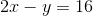?Explanation:

To solve this, first put the linear equation into slope-intercept form: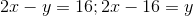.

Recall that in slope intercept form,

the m term is the slope value.

Therefore, the slope is 2.

### Example Question #22 : Graphing Linear Functions

How many-intercepts does the graph of the function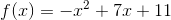have?

One

Zero

Two

Cannot be determined

Four

Two

Explanation:

The graph of a quadratic function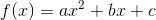has an-intercept at any point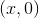at which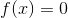, so, first, set the quadratic expression equal to 0: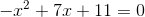The number of-intercepts of the graph is equal to the number of real zeroes of the above equation, which can be determined by evaluating the discriminant of the equation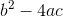. Set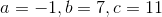, and evaluate: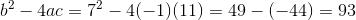The discriminant is positive, so there are two real solutions to the quadratic equation, and the graph of the function has two-intercepts.

### Example Question #11 : Graphing Inequalities

Which of the following graphs correctly depicts the graph of the inequalityExplanation:

Let's start by looking at the given equation: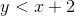The inequality is written in slope-intercept form; therefore, the slope is equal toand the y-intercept is equal to.

All of the graphs depict a line with slope ofand y-intercept. Next, we need to decide if we should shade above or below the line. To do this, we can determine if the statement is true using the origin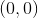. If the origin satisfies the inequality, we will know to shade below the line. Substitute the values into the given equation and solve.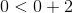Because this statement is true, the origin must be included in the shaded region, so we shade below the line.

Finally, a statement that is "less than" or "greater than" requires a dashed line in the graph. On the other hand, those that are "greater than or equal to" or "less than or equal to" require a solid line. We will select the graph with shading below a dashed line.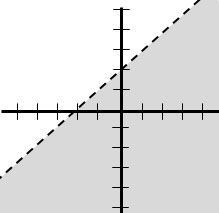1 3 Next →

### All Algebra II Resources